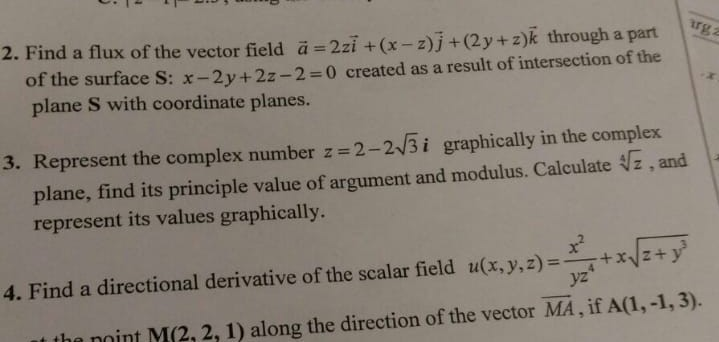Home / Answered Questions / Other / 2-find-a-flux-of-the-vector-field-a-2zi-x-z-j-2y-z-k-through-a-part-of-the-surface-s-x-2y-2z-2-0-cre-aw415

# (Solved): 2. Find A Flux Of The Vector Field Ã¤ = 2zi + (x - Z)j +(2y+z)k Through A Part Of The Surface S: X-2...2 and 3 with all steps

2. Find a flux of the vector field Ã¤ = 2zi + (x - z)j +(2y+z)k through a part of the surface S: x-2y + 2z-2=0 created as a result of intersection of the plane S with coordinate planes. 3. Represent the complex number z=2-223i graphically in the complex plane, find its principle value of argument and modulus. Calculate z , and represent its values graphically. +12+ Yz 4. Find a directional derivative of the scalar field u(x, y, z)= the point M(2,2,1) along the direction of the vector MA, if A(1,-1, 3).

We have an Answer from Expert